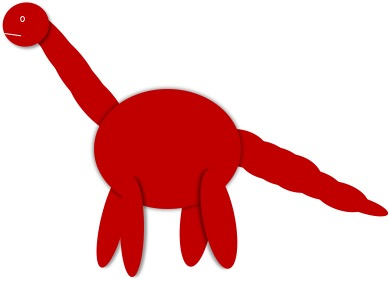# Instructions:

• This html will remind you of the code needed to do exploratory model selection
• It will have hints and suggests for the steps you need to do exploratory model selection
• Data will be named YourData and models YourModel - you should change these to the name you decide to give your data and models
• For this analysis you should choose whether to use the AIC or BIC and explain your choice.

The variable names are also different here as examples are on different data to what you have.

# Help and hints (and still some pictures)

Below are a list of click down sections, each covers a different part of the analysis. Use whichever you need. Also try to have a go on your own when you can, you can always check after if you got it right.

I expect you to use the R section for this type of model selection!

For this analysis we want to choose the ‘best’ model for fit and complexity. We want to try models with all of our explanatory variables in and then find the one that creates a balance between fit and complexity.

We can do this using the AIC or the BIC - you must choose which best fits your aims here.

Useful R code
# THIS EXAMPLE IS ON DIFFERENT DATA

# First create a null model with no variables:
# only the intercept
null <- lm(height ~ 1, data = YourData)
# Next create one model for each variable
model1 <- lm(height ~ type, data = YourData)
model2 <- lm(height ~ pair, data = YourData)
model3 <- lm(height ~ pot, data = YourData)

# Compare using the AIC and BIC
AIC(null, model1, model2, model3)
##        df      AIC
## null    2 157.5501
## model1  3 153.7789
## model2 16 175.1069
## model3  5 161.8181
BIC(null, model1, model2, model3)
##        df      BIC
## null    2 160.3525
## model1  3 157.9824
## model2 16 197.5260
## model3  5 168.8240

Remember: lower is better with AIC and BIC. Look at model formulas in lecture if you don’t remember.

The function bestglm() takes two main arguments: 1) a dataset with the response as the final column and IC which tells R the type of criteria to use for model selection e.g. “AIC” or “BIC”.

To use this we need to re-format our data so we have our response (y) as the final column in our dataset.

To start you will need to look at the order of the columns in your dataset. Then use something like the code below to re-order.

# Create a new dataframe with all explanatory columns first and
# the response last

# [row,column] these are ways of indexing inside your dataframe
# in this example we leave the row section blank to take all rows,
# we take columns 2 to 4 first and put column 1 (our response) at the end
NewData <- YourData[ ,c(2:4, 1)]

# now we can use bestglm()
OutputAIC <- bestglm(NewData, IC="AIC")
## Morgan-Tatar search since factors present with more than 2 levels.
OutputBIC <- bestglm(NewData, IC="BIC")
## Morgan-Tatar search since factors present with more than 2 levels.
coef(OutputAIC$BestModel) confint(OutputAIC$BestModel)

coef(OutputBIC$BestModel) confint(OutputBIC$BestModel)

OutputAIC$Subsets OutputBIC$SubsetsHints on which steps to include

Here, there are many models to compare.

First, think about what each of these individual models are.

Then decide how to compare them (there is a short way or a slower way - either is fine) and choose a statistic to use to compare them - justify your choice.

Then analyse the results.

I really don’t know which steps!! (or I just want to be sure)

The first step would be to calculate the AIC or BIC for all of the models with one explanatory variable and a null. So, that is a model for each explanatory variable and a null (Y~1).

Remember: lower is better with AIC and BIC. Look at model formulas in lecture if you don’t remember.

Doing this individually is great if you just want to test between single variables, but often we have more than one explanatory variable and we want to find the best model given a combination of these. I.e. our best model could have more than one variable in. You could test all of the possible combinations manually. But it is much faster to use a function.

The function bestglm(), is a good option. It takes two main arguments: 1) a dataset with the response as the final column and IC which tells R the type of criteria to use for model selection e.g. “AIC” or “BIC”.

Once you have the output from bestglm() you can see what it estimates the best model to be. You can also look this model in more detail using $BestModel (see below), which gives you the best model object. This using functions we are familiar with coef() and confint(). You can look at the AIC or BIC results for all of the trialled models using the outputobject$Subsets.How to read outputs of exploratory model selection
coef(OutputAIC$BestModel) confint(OutputAIC$BestModel)

coef(OutputBIC$BestModel) confint(OutputBIC$BestModel)

You can look at the AIC or BIC results for all of the trialled models using the outputobject$Subsets The ’best model is indicated with a * on the far left. Which variables were included is indicated by TRUE and FALSE and the loglikelihood and AIC or BIC are shown to the right. You might notice some AIC and BIC values are negative, on your own data. This is ok, we still want the lowest (most negative). OutputAIC$Subsets
##    Intercept   pot  pair  type logLikelihood      AIC
## 0       TRUE FALSE FALSE FALSE     -34.20690 68.41381
## 1*      TRUE FALSE FALSE  TRUE     -31.32127 64.64254
## 2       TRUE  TRUE FALSE  TRUE     -30.26492 68.52984
## 3       TRUE  TRUE  TRUE  TRUE     -24.71254 85.42508
OutputBIC\$Subsets
##    Intercept   pot  pair  type logLikelihood       BIC
## 0       TRUE FALSE FALSE FALSE     -34.20690  68.41381
## 1*      TRUE FALSE FALSE  TRUE     -31.32127  66.04374
## 2       TRUE  TRUE FALSE  TRUE     -30.26492  74.13463
## 3       TRUE  TRUE  TRUE  TRUE     -24.71254 110.64664